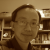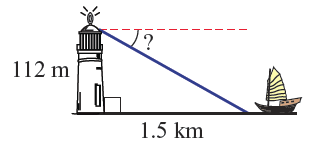# Sea## Sea simulationIn junior high school maths, there are usually questions about measuring the angle of depression and/or distance using trigonometry. Common examples could be like "Find the angle of depression from a lighthouse beacon 112 m above the sea level to a boat that is at a horizontal distance of 1.5 km from the lighthouse". A picture (not to scale) may be given as the one on the right. But then, what if the scenario is in 3D?## Waves at sea

Following my previous blog about 3D function graphing, I did another experiment to animate the 3D graphs. In this blog, I used the same 3D function f(x,z) = x*z^3-z*x^3 to generate two sets of heights. Then a VECTORINTERPOLATOR (or VECINT) was used to animate this 3D graph. It looks to me like a wave on the sea surface. What do you think?